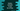# Python example to zip list of lists using zip method## Python example to zip list of lists using zip method:

We can pass any number of list items to the zip() method. This method can take multiple iterables. This method returns one iterator of tuples. Each tuple will have only one element if we pass one single list. For multiple lists, it will return an iterable of tuples. Each tuple will have elements from each lists.

We can also pass lists of different data types.

### Example program:

Let’s take a look at the below program:

``````first_list = [1,2,3,4]
second_list = ['first', 'second', 'third', 'fourth']
third_list = ['a', 'b', 'c', 'd']

zipped_result = zip(first_list,second_list,third_list)

print(set(zipped_result))``````

If you run this program, it will print the below output:

``{(1, 'first', 'a'), (3, 'third', 'c'), (4, 'fourth', 'd'), (2, 'second', 'b')}``

You can see that each tuple contains elements from each of these lists.

### Unzipping zipped values:

zip() can be used to unzip zipped values. For example:

``````first_list = [1,2,3,4]
second_list = ['first', 'second', 'third', 'fourth']
third_list = ['a', 'b', 'c', 'd']

zipped_result = zip(first_list,second_list,third_list)

unzipped_first_list, unzipped_second_list, unzipped_third_list = zip(*zipped_result)

print(unzipped_first_list)
print(unzipped_second_list)
print(unzipped_third_list)``````

It will print:

``````(1, 2, 3, 4)
('first', 'second', 'third', 'fourth')
('a', 'b', 'c', 'd')``````

Here, we are zipping the lists and unzipping the zipped content to three different lists.